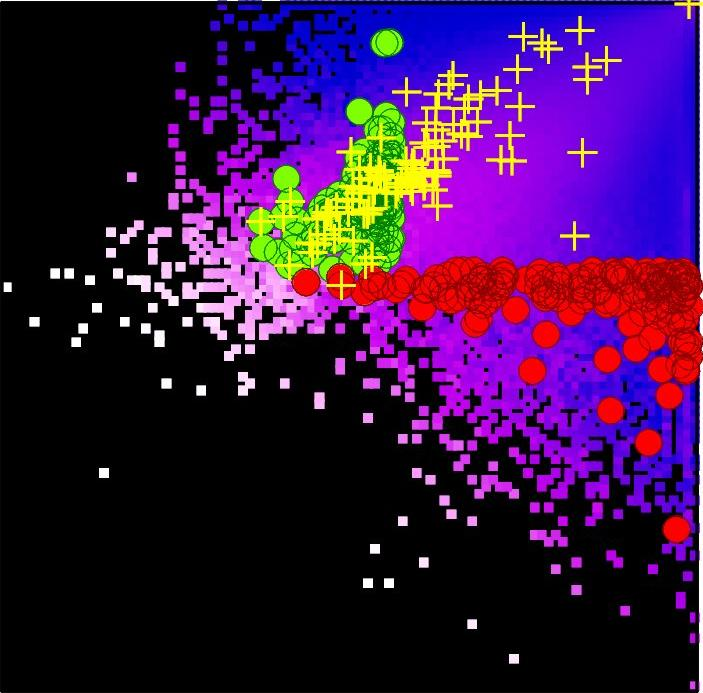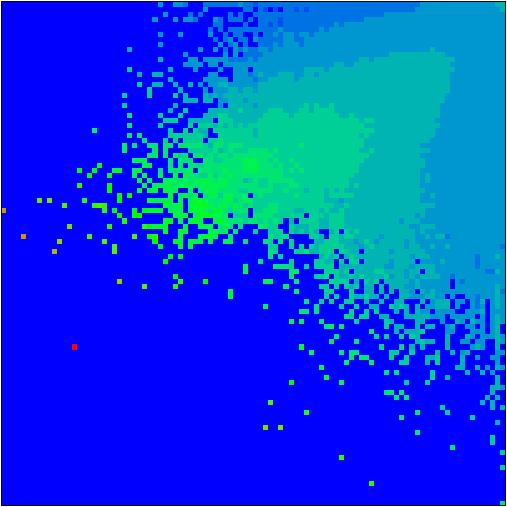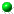Two-dimensional ranking of Wikipedia articles
by A.O.Zhirov, O.V.Zhirov and D.L.Shepelyansky
arXiv:1006.4270Density distribution of Wikipedia articles in 2DRank plane
(left: nodes in x-PageRank - y-CheiRank plane; center: green/red points show top 100 personalities in the plane, yellow pluses show top 100 personalities of Hart's book; right: right other color mode)

 ` `Arxiv preprint with minor corrections (here), updated version (here), EPJB published (here)All articles in PageRank order (file.gz 95MGb (here)), 2DRank order (here), CheiRank (here)L1) Top 100000 Wikipedia English articles in: PageRank (here), 2DRank (here), CheiRank (here)L2) Top 100 Wikipedia countries in: PageRank (here), 2DRank (here), CheiRank (here), SRJrank (here)L3) Top 100 Wikipedia universities in: PageRank (here), 2DRank (here), CheiRank (here), GoogleRank (here), Shanghairank(here)L4) Top 100 Wikipedia personalities in: PageRank (here), 2DRank (here), CheiRank (here), Hartrank (here)L5) Wikipedia ranking of physicists in: PageRank (here), 2DRank (here), CheiRank (here)L6) Wikipedia ranking of Nobel laureate physicists in: PageRank (here), 2DRank (here), CheiRank (here)L7) Wikipedia ranking of chess players in: PageRank (here), 2DRank (here), CheiRank (here)L8) Wikipedia ranking of Dow-Jones companies in: PageRank (here), 2DRank (here), CheiRank (here)L9) Local 2D Wikipedia ranking 100X100 on PageRank (x=K), CheiRank (y=K*) plane for (countries), (universities), (personalities), (physicists), (Nobel_physicists)L10) Source data with links and titles (321Mb) (here) This webpage is created at June 21, 2010 and is maintained by Dima Shepelyansky